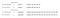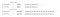# Floating-Point Representation in Java

Overview

Floating-point representation is used to represent non-integer fractional numbers in computer memory. The most commonly used floating-point representation is the IEEE-754 floating-point representation. IEEE-754 standard has 3 basic components.

• Sign bit: A single bit is allocated to represent the sign of the floating-point number.
• Exponent: A field of bits is allocated to represent both positive and negative exponents.
• Mantissa: A collection of bits are allocated to represent the decimal part of the floating-point number.

Floating-Point Representation in Java

In Java there are two primitive data types for floating-point numbers:

• float: Float data type is represented by 32-bits (4-bytes) in memory (by one word in JVM). The IEEE-754 standard representation of float data type is known as single-precision representation. Figure 1. shows the IEEE-754 standard representation of float data type in Java.
• double: This is represented by 64-bits (8-bytes) in memory (by two words in JVM). The IEEE-754 standard representation of this is known as double-precision representation. Figure 2. shows the IEEE-754 standard representation of double data type in Java.

The following explains the IEEE-754 standard representation using an example.

20: 10100

0.1: 0.00011001100110011001100110011001100110011…………

20.1: 10100.00011001100110011001100110011001100110011…………

20.1: 1.01000001100110011001100110011001100110011………… x 24

Example: Single-precision representation of 20.1.

Biased component: 127+4=131

131: 10000011

The IEEE 754 standard single-precision representation:

0 10000011 01000001100110011001100

Example: Double-precision representation of 20.1.

Biased component: 1023+4=1027

1027: 10000000011

The IEEE 754 standard double-precision representation:

0 10000000011 0100000110011001100110011001100110011001100110011001

Note: For sign bit, 0 is assigned for positive floating-point numbers and 1 is assigned for negative floating-point numbers. For exponent calculation, a bias is added to exponent as 127 for single precision and 1023 for double precision.

But when we consider Java, the standard representations of single and double precision in the memory are not the same as the exact ones we obtained above. Refer to figure 3.Figure 3. Exact and Rounding Representations of Single and Double Precision.

This is occurred due to the rounding error in java.

As per this, if the bit located after the final bit of mantissa is 1, 1 bit is added to the final bit of mantissa otherwise it remains the same. Refer to figure 4.Figure 4. Exact and Rounding Representations of Single and Double Precision in Detail.

As a solution for we can avoid this rounding error by using BigDecimal. Figure 5. shows the output difference obtained by using double data type and BigDecimal.

BigDecimal Class

BigDecimal is a Java class that extends java.lang.Number, declared in java.math package. This has defined methods to handle double and float data type variables with great precision. Here define some of the methods declared inside the BigDecimal class.

Converts arg1 `BigDecimal` to a `double`.

Converts arg1 `BigDecimal` to an `int`.

Converts arg1 `BigDecimal` to a `long`.

• arg1.equals`(Object x)`

Compares arg1 `BigDecimal` with the specified `Object` for equality.

• arg1.add`(BigDecimal arg2)`

Returns a `BigDecimal` whose value is `(arg1 + arg2).`

Converts arg1 `BigDecimal` to a `byte`.

• arg1.max`(BigDecimal arg2)`

Returns the maximum of arg1 `BigDecimal` and `arg2`.

• arg1.min`(BigDecimal arg2)`

Returns the minimum of arg1 `BigDecimal` and `arg2`.

• arg1.pow`(int n)`

Returns a `BigDecimal` whose value is arg1’s n th power.

• arg1.remainder`(BigDecimal divisor)`

Returns a `BigDecimal` whose value is `(`arg1`% divisor)`.

• arg1.divide`(BigDecimal divisor)`

Returns a `BigDecimal` whose value is `(`arg1`/ divisor).`

• arg1.multiply`(BigDecimal multiplicand)`

Returns a `BigDecimal` whose value is (arg1× multiplicand).

• arg1.subtract`(BigDecimal arg2)`

Returns a `BigDecimal` whose value is `(`arg1`- arg2).`

Returns the string representation of arg1 `BigDecimal.`

Conclusion

As per the things discussed above,

• Standard IEEE-754 representation is used to represent floating-point numbers.
• In Java due to the impact of rounding error, floating-point representations of double and float data types may differ from the exact representations.
• BigDecimal class is used to avoid this rounding error problem.

References

Associate Software Engineer, Undergraduate BSc (Hons) in Computer Science

## More from Hasini Sandunika Silva

Associate Software Engineer, Undergraduate BSc (Hons) in Computer Science